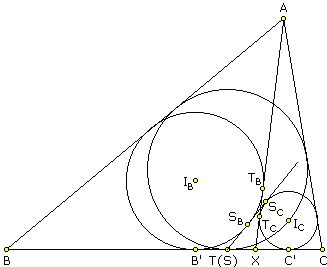# Three Incircles in a Triangle: What Is This About? A Mathematical Droodle

### This applet requires Sun's Java VM 2 which your browser may perceive as a popup. Which it is not. If you want to see the applet work, visit Sun's website at https://www.java.com/en/download/index.jsp, download and install Java VM and enjoy the applet.

 What if applet does not run?

ExplanationThis is an extension of another result concerning three incircles in a triangle.

### Theorem

Let the incircle of ΔABC touch the side BC at point T. Let X be another point on BC. Consider incircles of triangles ABX and ACX. Then the internal common tangent of the two circles other than AX, passes through T. Additionally, both X and T lie on a circle constructed on the segment joining the incenters of triangle ABX and ACX as diameter.

(For a partial history of the problem, a context and the credits, refer to D. Grinberg's post (Theorems 1 and 2) at the CTK Exchange.)

### Proof

Below, I shall refer to the following configuration, where X lies to the right of T.Let IB and IC be the incenters of triangles ABX and ACX, respectively; TB and TC are the points where their incircles touch AX. AX is thus an internal common tangent of the two circles. Assume their other internal common tangent SBSC crosses BC in point S. We want to show that S = T.

Obviously,

(1)

TBTC = SBSC.

If B' and C' are the points where the two incircles touch BC, then SB' = SSB and SC' = SSC, so that

(2)

SBSC = SSC - SSB = SC' - SB'.

On the other hand,

(3)

SBSC = TBTC = XTB - XTC = XB' - XC',

wherefrom

(4)

SB' + XB' = SC' + XC'.

And we conclude that the midpoints of segments SX and B'C' coincide, and therefore SB' = XC'. Now, as was shown by V. Zajic,

(5)

TX = TBTC.

From (3) and (5) we also have

(6)

TX + XC' = XB' = TB' + TX,

which implies XC' = TB', so that, finally, TB' = SB' and T = S.

Let's now consider the circle, say g, with diameter IBIC. IB lies on the bisector of angle BXA, while IC lies on the bisector of the supplementary angle CXA. Since the two bisectors are orthogonal, X lies on g. Since TSBSC is also tangent to the two circles, IB and IC lie on the bisectors of BTSBSC and CTSBSC - another pair of supplementary angles. The two are again orthogonal, and therefore T, too, lies on g.